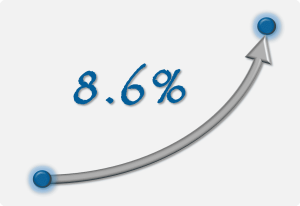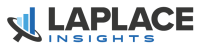# Annualized Return or CAGRThe Annualized Total Return, also called the Compounded Annual Growth Rate (CAGR), is a useful number to describe the performance of an investment.  Never confuse this with Annual Returns, which is a bunch of numbers that show the returns of an investment for each year during the investment time frame.  Contrary to this, the annualized return is a single number that represents the investment return over the entire time frame.

In other words, the annualized return is a single number that measures the performance from the beginning of the investment time frame all the way to the end of the time frame.  We just calculate the compounded rate of return between these two endpoints.

This implies two important things:

1. Anything that happened between these two dates does not affect the annualized return number and,
2. The number is a compounded figure, which means that all investment profits and losses are assumed to be accumulated over the time frame, irrespective of when they happened.

## A Simple Annualized Return Example

Let’s consider a simple example to illustrate.

Suppose you analyze the price of a stock during a 10-year time frame, from January 2, 2004, until December 31, 2013.  Suppose the stock opened at \$25.00 on January 2, 2004, and closed at \$50.00 on December 31, 2013.  The CAGR, or Compounded Annual Rate of Return, corresponds to the average constant annual rate of return you need to grow the \$25 to \$50 during the 10-year time frame.  The formula to calculate it is this:In our example, the Final Value is \$50, the Starting Value is \$25, and N is 10 years, so our CAGR is 7.2%

Observe that the stock could have grown steadily from \$25 to \$50 to give us this return over the period.  Or, it could have given us a crazy wild ride.  For example, it may have crashed to \$2 during the financial crisis, go nowhere until 2012, and finally, through a wild and massive upswing, come all the way back and close 2013 at \$50.

In both cases, the annualized return is identical but the volatility is drastically different.  More specifically the standard deviation and the maximum drawdown are completely different stories.  So even though the annualized return is an important figure, it represents only one aspect of performance.  Risk is the other side of the story.  Risk is often overlooked, but it is arguably the most important measure you need to understand so you can make sure you make money consistently.# Global Allocation Research Report, August 2020 Edition

Below you will find the Columbus strategy for this month. For

# Global Allocation Research Report, July 2020 Edition

Below you will find the Columbus strategy for this month. For